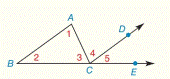Chapter 2.CR, Problem 16CR### Elementary Geometry for College St...

6th Edition
Daniel C. Alexander + 1 other
ISBN: 9781285195698

#### Solutions

Chapter
Section### Elementary Geometry for College St...

6th Edition
Daniel C. Alexander + 1 other
ISBN: 9781285195698
Textbook Problem
1 views

# Given: m ∠ 1 = x 2 − 12 m ∠ 4 = x ( x − 2 ) Find: x so that A B ¯ ∥ C D →To determine

To find:

The value of x.

Explanation

Given:

The given statement is,

m1=x212m4=x(x2)AB¯CD

Figure (1)

Concept:

If two parallel lines are cut by a transversal, then a pair of alternate interior angle is congruent.

Calculation:

The given statement is,

m1=x212m4=x(x2)AB¯CD

1 and 4 are alternate interior angles

### Still sussing out bartleby?

Check out a sample textbook solution.

See a sample solution

#### The Solution to Your Study Problems

Bartleby provides explanations to thousands of textbook problems written by our experts, many with advanced degrees!

Get Started

#### Find more solutions based on key concepts